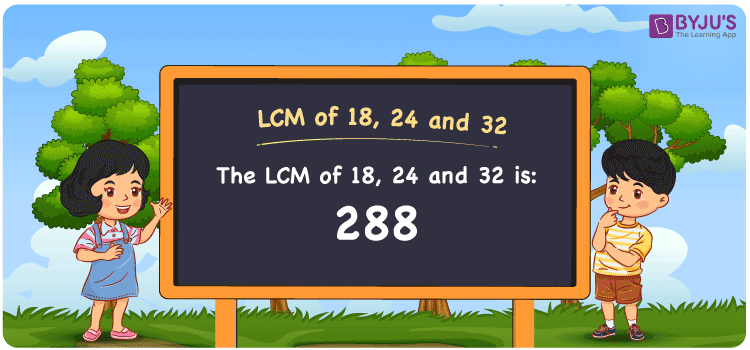# LCM of 18, 24 and 32

LCM of 18, 24 and 32 is 288. The least common multiple of two numbers is the smallest number which is a multiple of both the numbers. The article LCM of Two Numbers designed by expert faculty in a simple language is the best study guide to clear the doubts of students instantly. Referring to this article while solving problems helps students to understand the LCM concept in a better way. Let us glance at how to find the least common multiple of 18, 24 and 32 in a detailed manner in this article.

## What is LCM of 18, 24 and 32?

The answer to this question is 288.## How to Find LCM of 18, 24 and 32?

The LCM of 18, 24 and 32 can be obtained using three methods:

• Prime Factorisation
• Division method
• Listing the multiples

### LCM of 18, 24 and 32 Using Prime Factorisation Method

The prime factorisation method is one of the most common ways of determining LCM. To calculate the LCM of 18, 24 and 32, we use the steps as follows:

18 = 2 × 3 × 3

24 = 2 × 2 × 2 × 3

32 = 2 × 2 × 2 × 2 × 2

LCM (18, 24, 32) = 2 × 2 × 2 × 2 × 2 × 3 × 3 = 288

### LCM of 18, 24 and 32 Using Division Method

In this method, we divide the numbers 18, 24 and 32 by their prime factors until we get the result as one in the complete row to find their LCM. The product of these divisors gives the least common multiple of 18, 24 and 32.

 2 18 24 32 2 9 12 16 2 9 6 8 2 9 3 4 2 9 3 2 3 9 3 1 3 3 1 1 x 1 1 1

No more further division can be done.

Hence, LCM (18, 24, 32) = 2 × 2 × 2 × 2 × 2 × 3 × 3 = 288

### LCM of 18, 24 and 32 Using Listing the Multiples

In this method, the multiples of each number are listed until the first common multiple is found. The multiples of 18, 24 and 32 are given below:

Multiples of 18: 18, 36, 54, 72, 90, 108, 126, 144, 162, 180, ……., 252, 270, 288, ……….

Multiples of 24: 24, 48, 72, 96, 120, 144, 168, 192, 216, 240, ….., 240, 264, 288, ……..

Multiples of 32: 32, 64, 96, 128, 160, 192, 224, 256, 288, ………….

LCM (18, 24, 32) = 288

## Related Articles

Least Common Multiple (LCM)

LCM calculator

Prime Factorization and Division Method for LCM and HCF

LCM Formula

LCM with Examples

HCF and LCM

## Video Lesson on Applications of LCM## Solved Example

Question: What is the smallest number that is divisible by 18, 24, 32 exactly?

Solution: The smallest number that is divisible by 18, 24, 32 exactly is their LCM. The LCM of 18, 24 and 32 is 288. Hence the smallest number that is divisible by 18, 24, 32 exactly is 288.

## Frequently Asked Questions on LCM of 18, 24 and 32

### What is the LCM of 18, 24 and 32?

The LCM of 18, 24 and 32 is 288.

### Is the LCM of 18, 24, and 32 the same as the HCF of 18, 24 and 32?

No. The LCM of 18, 24, and 32 is 288 and the HCF of 18, 24 and 32 is 2.

### Is 188 the LCM of 18, 24 and 32?

No. The LCM of 18, 24 and 32 is 288.

### Name the methods used to find the LCM of 18, 24 and 32.

The following methods are used to find the LCM of 18, 24 and 32 are:

Prime Factorisation

Division Method

Listing the Multiples

### How to find the LCM of 18, 24 and 32 using Prime Factorisation?

18 = 2 × 3 × 3

24 = 2 × 2 × 2 × 3

32 = 2 × 2 × 2 × 2 × 2

LCM (18, 24, 32) = 2 × 2 × 2 × 2 × 2 × 3 × 3 = 288

Thus the LCM of 18, 24 and 32 using Prime Factorisation is 288